# Clock using Arduino UNO, 16 x 2 LCD Display, and Switches

My research project on microcontrollers, using a Arduino UNO R3 and other components.

IntermediateWork in progress606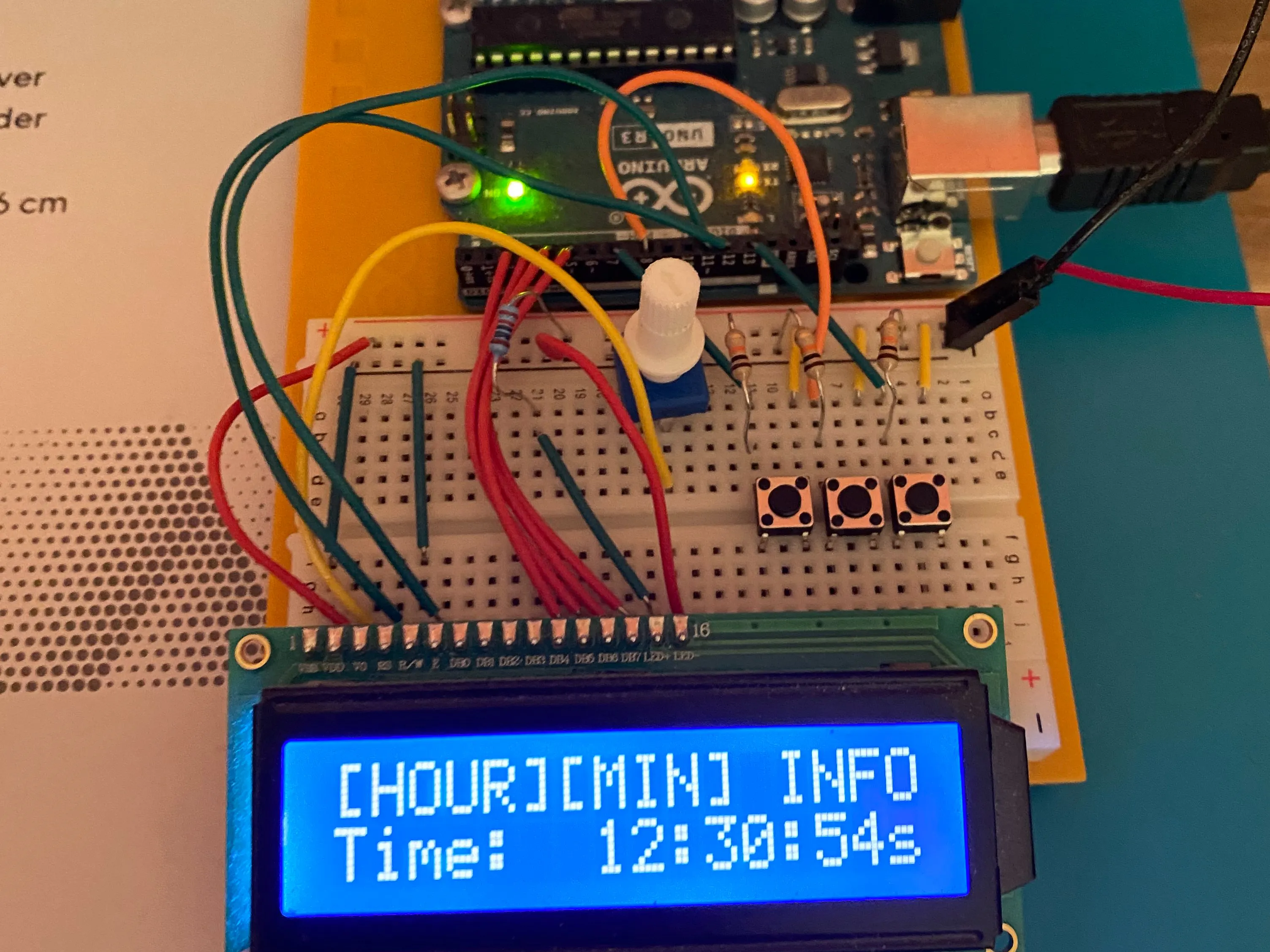## Things used in this project

### Hardware components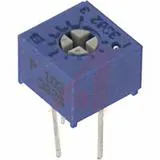Single Turn Potentiometer- 10k ohms
×1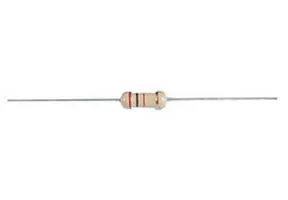Resistor 220 ohm
×1Resistor 10k ohm
×3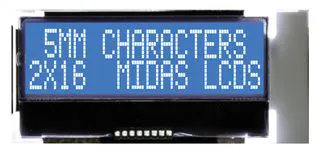Alphanumeric LCD, 16 x 2
×1Arduino UNO
×1SparkFun Pushbutton switch 12mm
×3

## Schematics

### Use project 9 and 11 from projects book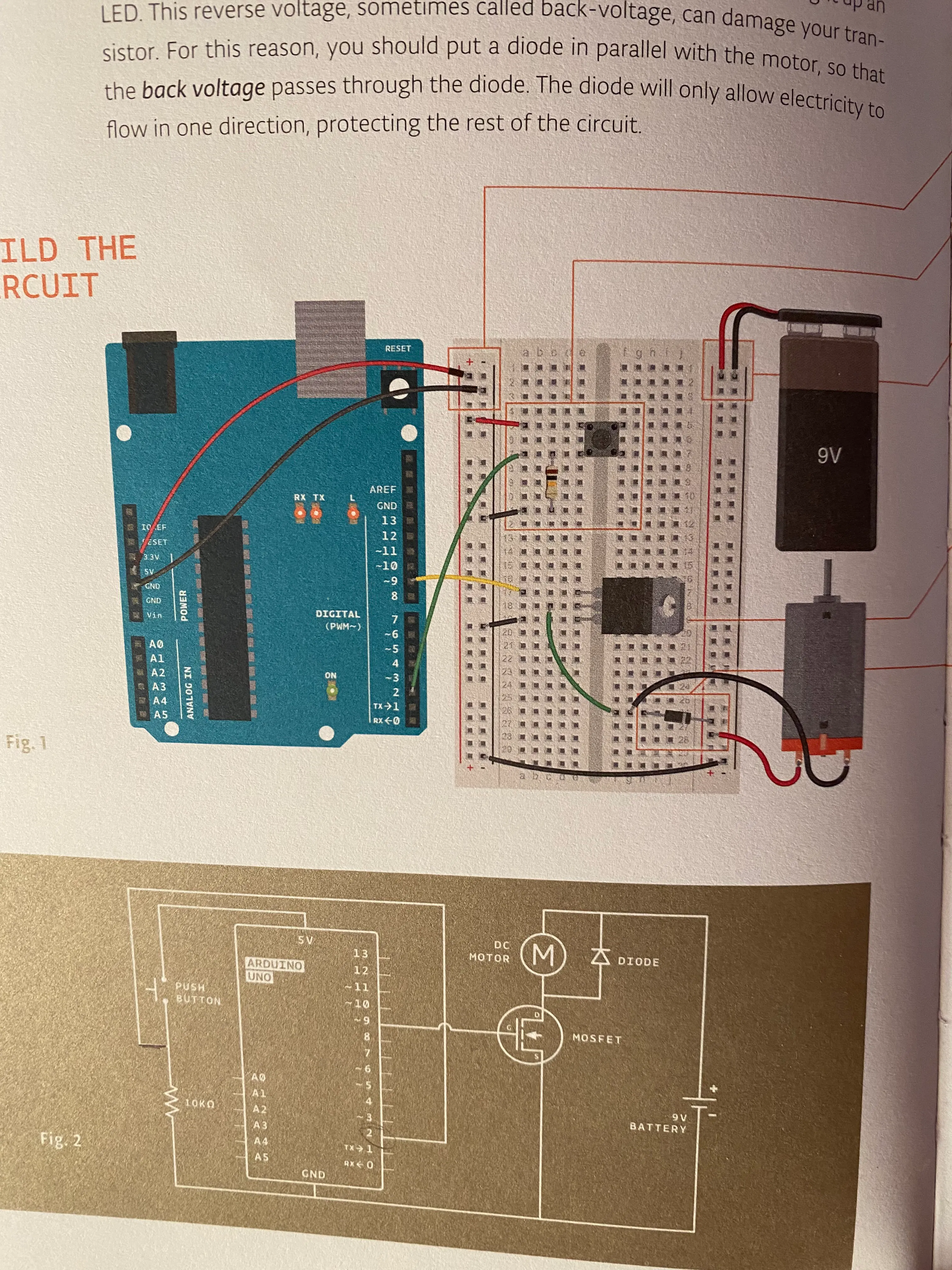### Replace the tilt sensor in project 11 with the switch from project 9

Looking at the code, the way the button works in project 9 will allow you to use a switch, for this project the switch will adjust hour and minutes.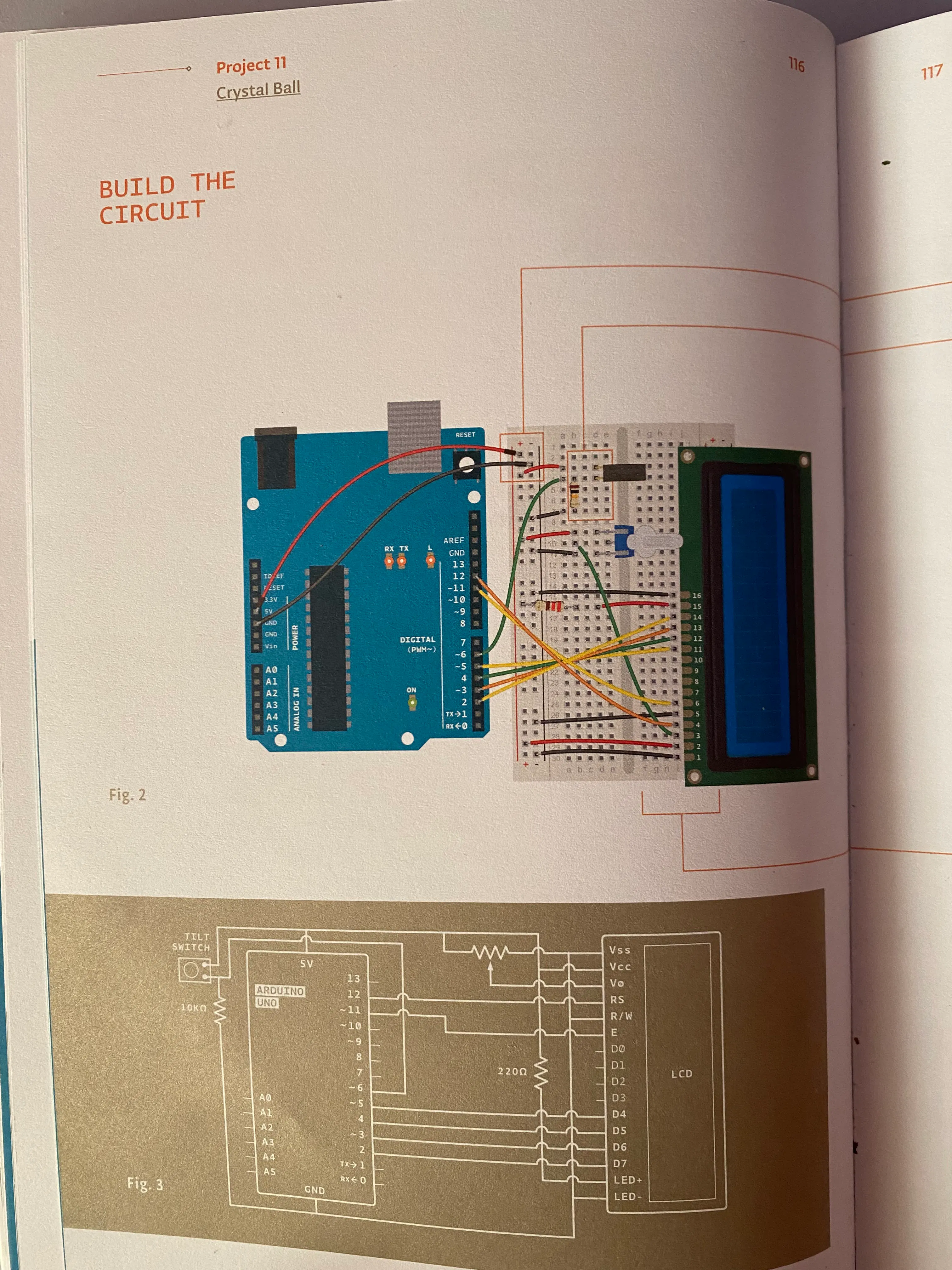## Code

### Code for Arduino

Arduino
This code allows the clock to constantly count seconds while allowing you to press buttons to change the hour or the minute.
```unsigned long seconds;
int hour = 1; // what hour the program starts with
int minute = 0;

#include <LiquidCrystal.h>
LiquidCrystal lcd(12,11,5,4,3,2);

const int pinone = 8; // the pin that the arduino is reading
const int pintwo = 13; // the pin that the arduino is reading
const int pinthree = 7; // the pin that the arduino is reading
int state = 0;
int statetwo = 0;
int statethree = 0;

void setup() {
// put your setup code here, to run once:
Serial.begin(9600);

pinMode(pinthree,INPUT);

lcd.begin(16, 2);
lcd.setCursor (0, 0);
lcd.print("This is a clock");
lcd.setCursor (0, 1);
lcd.print("Time:");
seconds = 0;

}

void loop() {

// put your main code here, to run repeatedly:
Serial.print("Time: ");

Serial.println(seconds);
delay(1000); // 1000 milliseconds = 1 second
seconds=seconds+1;

lcd.setCursor(13,1);
lcd.print(seconds); // display seconds
lcd.print("s");  // 1 second passes

lcd.setCursor(10,1);
lcd.print(minute); // display minutes
lcd.print(":");

lcd.setCursor(7,1);
lcd.print(hour); // display hour
lcd.print(":");

if(statethree == HIGH){ // When switch is pressed it will read HIGH

hour++; // LEFT BUTTON - add a hour

lcd.setCursor(0,0);
}
else if(state == HIGH){ // When switch is pressed it will read HIGH

minute++; //MIDDLE BUTTON - add a minute

lcd.setCursor(0,0);
}

else if (statetwo == HIGH){ // When switch is pressed it will read HIGH

lcd.setCursor(0,0);
lcd.print("[HOUR][MIN] INFO"); //RIGHT BUTTON - show info
}

if (seconds >= 60){
seconds = 0;
minute = minute + 1;  // count up to a minute

if (minute >= 60){
minute=0;
hour = hour + 1; // count up to a hour

if (hour == 13){ // clock does not pass 12, after 12, the next number should be 1
hour = 1;

}
}
}
}
```

## Credits

### jackycppp

0 projects • 0 followers